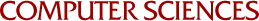## Solution of Symmetric Linear Complementarity Problems by Iterative Methods

Olvi Mangasarian
1976

A unified treatment is given for iterative algorithms for the solution of the symmetric 1inear complementarity problem: Mx + q > 0, x > 0, xT(Mx+q) = 0, where M is a given nxn symmetric real matrix and q is a given nxl vector. A general algorithm is proposed in which relaxation may be performed both before and after projection on the nonnegative orthant. The algorithm includes as special cases, extensions of the Jacobi, Gauss-Seidel and nonsymmetric and symmetric successive over-relaxation methods, for solving the symmetric linear complementarity problem. It is shown first that any accumulation point of the iterates generated by the general algorithm solves the 1inear complementarity problem. It is then shown that a class of matrices for which the existenceof an accumulation point that solves the linear complementarity problem is guaranteed, is the class of symmetric copositive plus matrices which satisfy a qualification of the type: Mx + q > 0 for some x in Rn. This class includes symmetric positive semidefinite matrices satisfying this qualification, symmetric strictly copositive matrices and symmetric positive matrices.